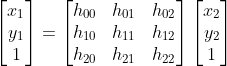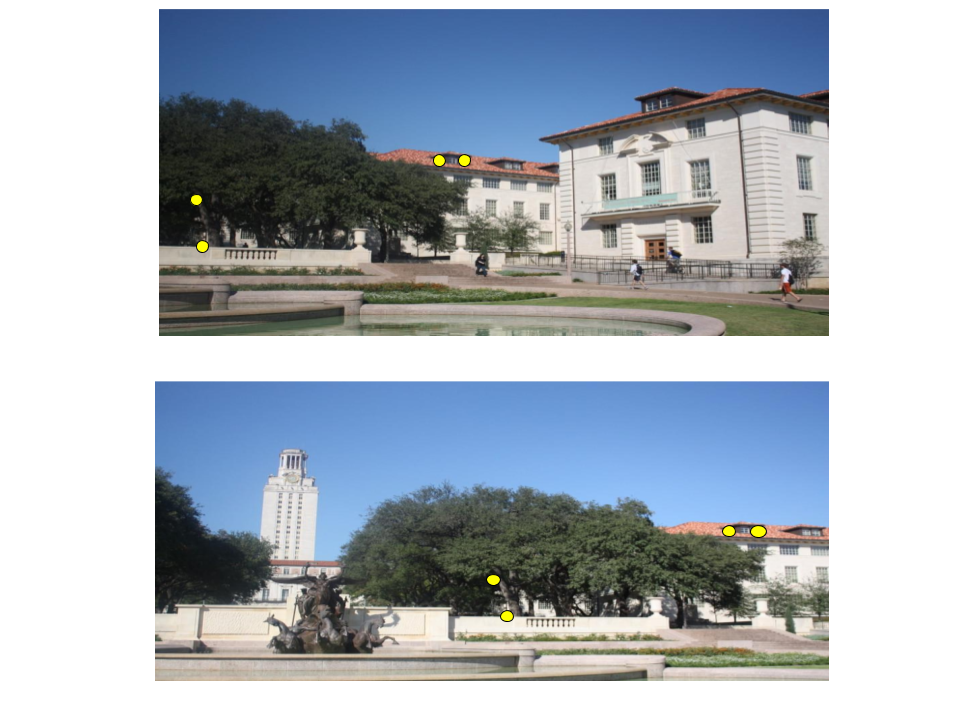# Finding Homography Matrix

It’s time to align the images now.

We need to align the images because, though both images could form a panorama, the two could differ in terms of angle, translation, size, etc, probably caused due to orientational difference in the camera while capturing the photos. Thus, we need to find a transformation matrix to perform this alignment, which ensures compatibility to stitch and form a panorama.

A Homography Matrix is a 3x3 transformation matrix that maps the points in one image to the corresponding points in the other image.where (x1, y1) and (x2, y2) could be thought of the co-ordinates of the matching key-points in both the images respectively.

A homography matrix is used with the best matching points, to estimate a relative orientation transformation within the two images. Simply put, it is the perspective transformation matrix between two planes - here, the two images.

In order to find a homography matrix, at least 4 matching key-points are needed.Note:

`cv2.findHomography` finds a perspective transformation between two planes - here, the two images. More here.

`cv2.RANSAC` is a method used to compute a homography matrix.

INSTRUCTIONS
• Get the best matches - `matches` - between the right and left images. If there are at least 4 matches, find the Homography matrix. Else, raise an error.

``````if len(matches) >= 4:
src = np.float32([ kp1[m.queryIdx].pt for m in matches ]).reshape(-1,1,2)
dst = np.float32([ kp2[m.trainIdx].pt for m in matches ]).reshape(-1,1,2)

H, masked = cv2.findHomography(src, dst, cv2.RANSAC, 5.0)
else:
raise AssertionError("Can't find enough keypoints.")
``````

No hints are availble for this assesment

Answer is not availble for this assesment

Note - Having trouble with the assessment engine? Follow the steps listed here